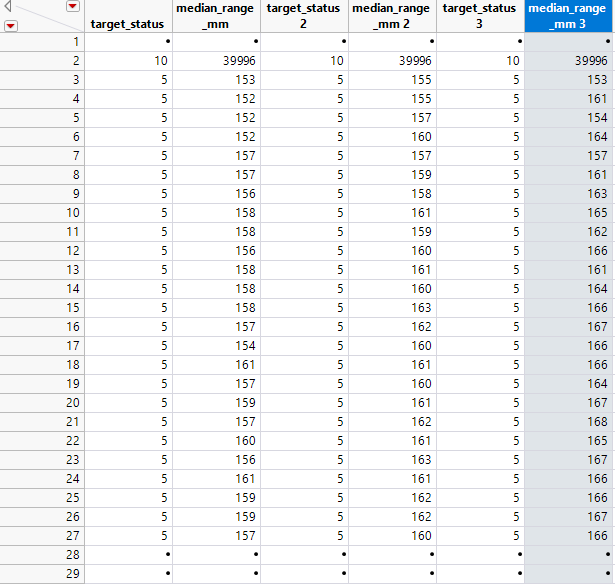Choose Language Hide Translation Bar
Highlighted

## How to use max among several column with "get rows where" condition

It seems an easy question but I'm struggling to solve it...

I have this kind of data table  :It goes until 64 which is the parameter "dim" in my script.

I'm looking for the maximum and minimum of all median_range_mm 1, 2 , 3 ... until 64. But I don't want JMP to look at rows where target_status is different of 5 or 9.

As you can see, when target status is 10 it overranges at 39996.

It is easier to understand with my code  :

``````maxValueVar = 0 ;
maxValue = Max( :median_range_mm [dt << get rows where( :target_status == 5 | :target_status == 9 ) ] );
minValue = Min( :median_range_mm[dt << get rows where( :target_status == 5 | :target_status == 9 )] );
For( p = 2, p <= dim, p++,
name = Column(dt, "target_status " || Char( p ));
nom = Column(dt, "median_range_mm " || Char( p )); // browse every columns of median_range_mm
MaxValueVar =  Col Max( nom [dt << get rows where ( name == 5 | name == 9 )] );

If(
maxValueVar > maxValue, maxValue = maxValueVar,
minValueVar < minValue, minValue = minValueVar
);
);``````

Notice that It works for the first column that I didn't parametered. Also It works if I comment

``[dt << get rows where ( name == 5 | name == 9 )]`   `

Any idea of how "get rows where" or "column max () " work ?

Thanks

3 REPLIES 3
Highlighted

## Re: How to use max among several column with "get rows where" condition

@Rob128,
I am struggling to understand exactly what you want ? Let me try and show you some steps:

The following should give you all the rows, where target_status is either 5 or 9, like you have tried.

``SR = dt << Select Where(:target_status ==5 | :target_status ==9) << get selected rows; ``

Now, you have the list of rows where your condition is met, the next thing you want if I understand your question is the min and macimum value ?

``````MedianValues = dt:median_range_mm << Get Values;

MedianValuesDes = MedianValues[SR]; // Filter out and get only the rows with target status of 5 or 9

MaxVal = Max(MedianValuesDes );
MinVal = Min(MedianValuesDes ); ``````

I am pretty sure there are many more ways to do it, but I am trying to break it down into steps so you can follow the thought. If this is not what you are after, can you explain a little more clearly what you are after please  ?

Best
Uday
Highlighted

## Re: How to use max among several column with "get rows where" condition

@txnelson

@uday_guntupalli

Thank you for your answers. Maybe I wasn't clear enough in stating my problem indeed.

The main blocking point is that I can get the Maximum of the column "median_range_mm" with the filter on the column "target_status" with code :

``````maxValue = Max( :median_range_mm [dt << get rows where( :target_status == 5 | :target_status == 9 ) ] ); // ==> OK
minValue = Min( :median_range_mm[dt << get rows where( :target_status == 5 | :target_status == 9 )] ); // ==> OK``````

But once I iterate over columns :

`For( p = 2, p <= dim, p++, name = Column("target_status " || Char( p )); nom = Column("median_range_mm " || Char( p )); MaxValueVar = Max( nom [dt << get rows where ( name == 5 | name == 9 )] );`

MaxValueVar and MinValueVar get no data "." (I guess it is Missing Value character for numeric columns) .

On debugging window I think the type of "name" and "nom" matters. It's not a column type...

The idea is that "name" is the column "target_status 2" then 3 then 4 ... with iteration on p.

Same idea with "nom" which is the column "median_range_mm 2" then 3 then  4 ....

So for p = 2,  I'm looking for the maximum of median_range_mm 2 where "target_status 2" == 5 or 9.

Then p = 3 ... until 64

Finally, if "maximum of median_range_3" > "maximum of median_range_2" then "maximum of median_range_3 takes the maxValue :

``````If(
MaxValueVar > maxValue, maxValue = MaxValueVar,
MinValueVar < minValue, minValue = MinValueVar
);``````

Highlighted

## Re: How to use max among several column with "get rows where" condition

I think this might be a solution for what you are attempting to do

``````min_value = Col Quantile( If( Row() <=64 & ( :target_status == 5 |
:target_status == 9 ),
:median_range_mm
,
.
), .5);``````
Jim
Article Labels

There are no labels assigned to this post.